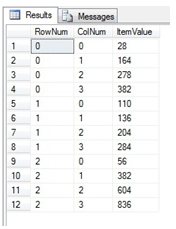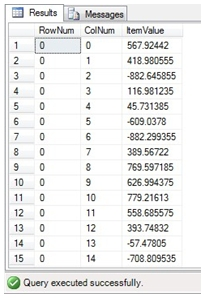# SQL Server MMULT function

MMULT_q

Updated: 17 August 2010

Use MMULT_q to calculate the matrix product of two arrays. The result is a TABLE where the maximum row number is equal to the number of rows in the first array and the maximum column number is the number of columns in the second array

For matrices in normalized form, use the MMULTN_q function.
Syntax
SELECT * FROM [wctMath].[wct].[MMULT_q] (
<@Matrix_A_RangeQuery, nvarchar(max),>
,<@Matrix_B_RangeQuery, nvarchar(max),>)
Arguments
@Matrix_A_RangeQuery
the select statement, as text, used to determine the ‘A’ matrix to be used in this function. When selecting data from a TABLE or a VIEW, specify the column names. If you just want to pass the values directly to the function, you can simply specify the values in the SELECT statement and use UNION ALL to enter the next row(s). Make sure that the entered values are of the type float or of a type that implicitly converts to float. Data returned from a TABLE or a VIEW in the @Matrix_A_RangeQuery select must be of the type float or of a type that implicitly converts to float.
@Matrix_B_RangeQuery
the select statement, as text, used to determine the ‘B’ matrix to be used in this function. When selecting data from a TABLE or a VIEW, specify the column names. If you just want to pass the values directly to the function, you can simply specify the values in the SELECT statement and use UNION ALL to enter the next row(s). Make sure that the entered values are of the type float or of a type that implicitly converts to float. Data returned from a TABLE or a VIEW in the @Matrix_B_RangeQuery select must be of the type float or of a type that implicitly converts to float.

Return Types
TABLE (
[RowNum] [int] NULL,
[ColNum] [int] NULL,
[ItemValue] [float] NULL
Remarks
·         The number of columns in the ‘A’ array must be equal to the number of rows in the ‘B’ array or an error will be returned.
·         Use the MMULT function for simpler queries.
·         Use MMULTN_q for a table in normal form.
·         If the array contains NULL, then NULL will be returned.
·         If the array contains a blank, it will be treated as zero.
·         The function returns an error if either array contains a non-numeric value.
Examples
In this example, we calculate the matrix product directly from the SELECT statement.
SELECT C.*
FROM wct.MMULT_q(
'SELECT 5,6,7,8 UNION ALL
SELECT 9,10,-11,12 UNION ALL
SELECT 16,15,14,13',
'SELECT 1,8,9,13 UNION ALL
SELECT 2,7,10,14 UNION ALL
SELECT -3,6,11,15 UNION ALL
SELECT 4,5,12,16'
) C

This produces the following resultHere’s another example, where we want to take a table that has 51 columns of data and we want to select the values in those columns and increase them 5%. You could to this by constructing a (very long) SELECT statement where you simply Select the column * 1.05. However, by using some other XLeratorDB functions, we can get the column names, put them in a string variable and pass that information to the MMULT_q function.

--Create a variable to store the SELECT statement for
--the 'B' side of the multiplication
DECLARE @SQLSTRING nvarchar(max)

--Populate the variable
SET @SQLSTRING = (Select 'select '
--use the JOINSTR_q function to concatenate
--the column names
+ wct.JOINSTR_q (',' + wct.CRLF()
,null
--Get the column information from the table
,'SELECT
COLUMN_NAME
FROM information_schema.columns
WHERE TABLE_NAME = ''MATRIX_D''
AND COLUMN_NAME like ''col%''')
--add the WHERE Clause to the SELECT
+ wct.CRLF() +
'FROM MATRIX_D
WHERE MATRIXNO = 1046
AND MATRIXID = ''B''
AND ROWNO = 1')

--Get the matrix product
SELECT C.*
FROM wct.MMULT_q(
'SELECT 1.05',
@SQLSTRING
) C

Here’s a snaphot of the part of the result.Copyright 2008-2023 Westclintech LLC         Privacy Policy        Terms of Service